# Perimeter Of Compound Shapes

Here we will learn about the perimeter of compound shapes, including how to find the perimeter of compound shapes.

There are also perimeter of compound shapes worksheets based on Edexcel, AQA and OCR exam questions, along with further guidance on where to go next if you’re still stuck.

## What is perimeter of compound shapes?

Perimeter of compound shapes is the distance around a compound shape.

The perimeter of a shape is finding the total distance around the outside of a 2D shape.

A compound shape (or composite shape) is any shape which is made up of two or more shapes. They are often rectilinear shapes which have straight sides and right angles.

For example,

Find the perimeter of this compound shape.

To find the perimeter we add all the lengths of the sides.

3+2+4+3+7+5=24

The perimeter is 24 \ cm.

### What is perimeter of compound shapes?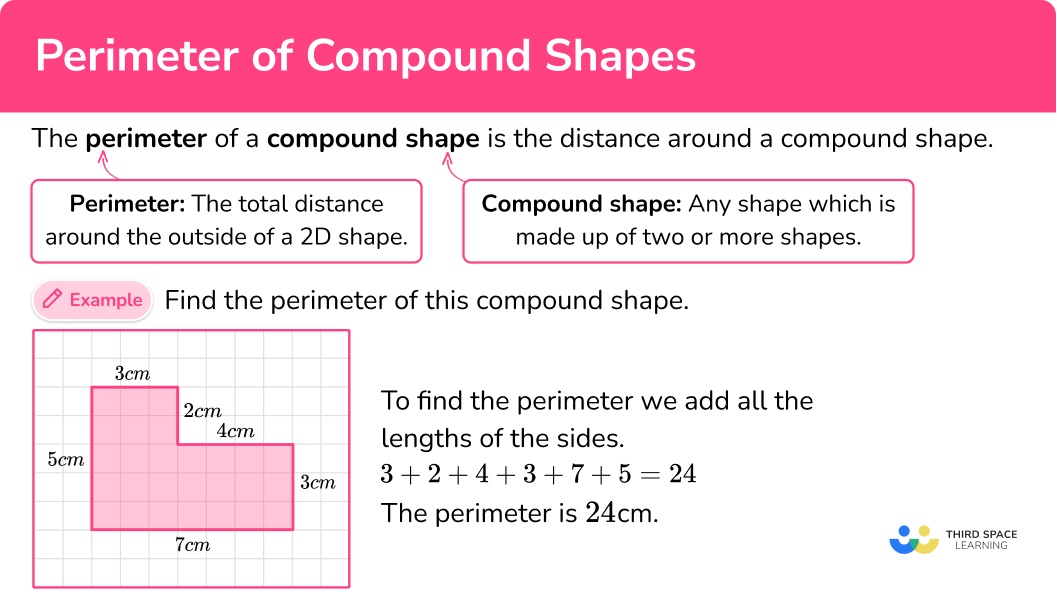## How to calculate the perimeter of compound shapes

In order to calculate the perimeter of compound shape:

1. Work out any missing lengths.
2. Add all the side lengths.
3. Write the final answer with the correct units.

### Explain how to calculate the perimeter of compound shapes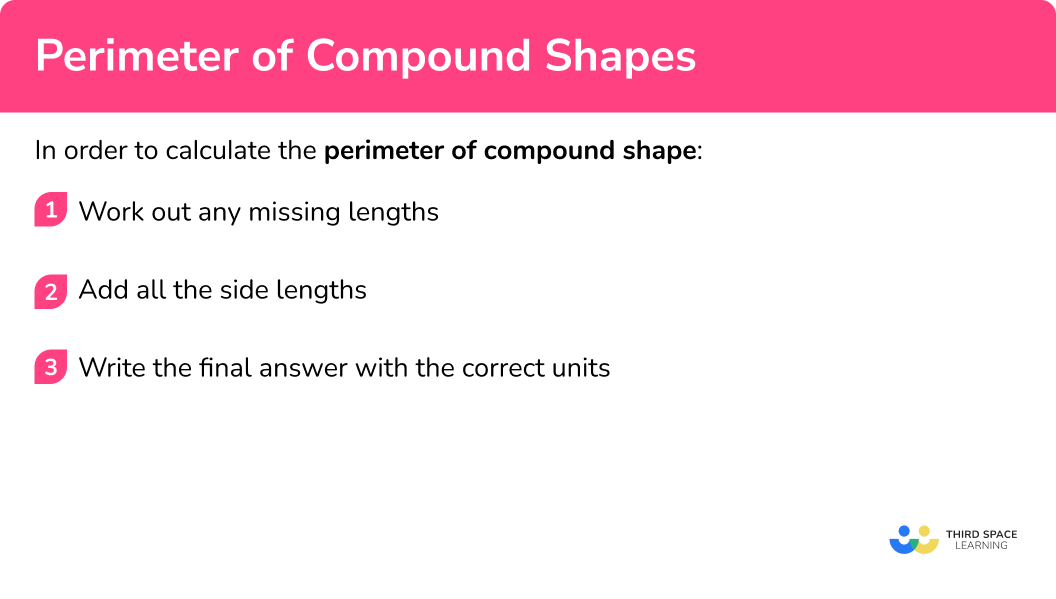### Related lessons on how to work out perimeter

Perimeter of compound shapes is part of our series of lessons to support revision on 2D shapes and how to work out perimeter. You may find it helpful to start with the main 2D shapes lesson for a summary of what to expect, or use the step by step guides below for further detail on individual topics. Other lessons in this series include:

## Perimeter of compound shapes examples

### Example 1: rectilinear shapes

Calculate perimeter.

1. Work out any missing lengths.

There are some missing lengths we need to work out first. We can look at the vertical lengths. The missing vertical length is 7 \ cm because 11-4=7.

We can then look at the missing horizontal length. The missing side is 5 \ cm because 7-2=5.

Starting in the top left corner and going clockwise around the shape, we can add the sides together.

2+7+5+4+7+11=36

3Write the final answer with the correct units.

The perimeter of the compound shape is 36 \ cm.

### Example 2: rectilinear shapes

Calculate perimeter.

There are some missing lengths we need to work out first. We can look at the vertical lengths. The missing vertical lengths are 6 \ cm and 13 \ cm.

We can then look at the missing horizontal length. The missing side is 16 \ cm because 7+5+4=16.

Starting in the top left corner and going clockwise around the shape, we can add the sides together.

16+13+4+6+5+6+7+13=70

The perimeter of the compound shape is 70 \ cm.

### Example 3: finding the missing side given the perimeter

The perimeter is 58.6 \ m, find x.

We can work out the missing horizontal length. The missing side is 11.9 \ m because 4.1+7.8=11.9.

We still don’t know the missing vertical length. We can write it as x+10.3.

Starting in the bottom left corner and going clockwise around the shape, we can add the sides we have together.

x+7.8+10.3+4.1+(x+10.3)+11.9

This algebraic expression simplifies to

2x+44.4.

We can then use the information that the perimeter is 58.6 \ m to form an equation and solve it to find x.

\begin{aligned} 2x+44.4 &= 58.6 \\\\ 2x &= 14.2 \\\\ x&=7.1 \end{aligned}

The perimeter of the compound shape is 58.6 \ m. The missing side is 7.1 \ m, so x=7.1.

### Common misconceptions

• Order of adding the sides

Addition is commutative. This means it doesn’t matter what order the sides of the shape are added in.

• Check the units

Check which units are involved in the question as there may be a mixture.

### Practice perimeter of compound shapes questions

1. Find the perimeter of this 2D shape.31 \ cm30 \ cm39 \ cm38 \ cmThe perimeter is 38 \ cm because

4+2+7+8+11+6=38.

2. Find the perimeter of this 2D shape.23 \ cm25 \ cm28 \ cm20 \ cmThe perimeter is 28 \ cm because

5+1+4+3+7+8=28.

3. Find the perimeter of this 2D shape.33 \ m44 \ m78 \ m148 \ mThe missing sides are 5 \ m and 6 \ m.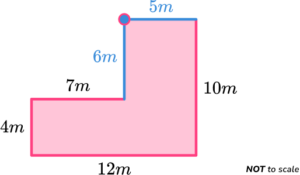The perimeter is 44 \ m because

5+10+12+4+7+6=44.

4. Find the perimeter of this 2D shape.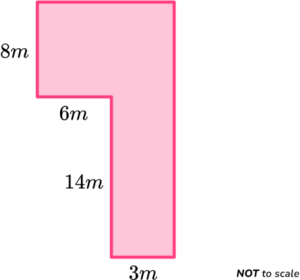31 \ m114 \ m90 \ m62 \ mThe missing sides are 9 \ m and 22 \ m.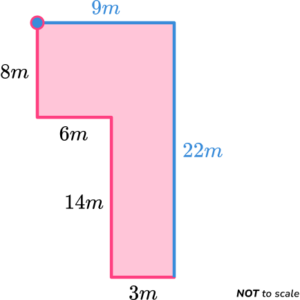The perimeter is 62 \ m because

9+22+3+14+6+8=62.

5. Find the perimeter of this 2D shape.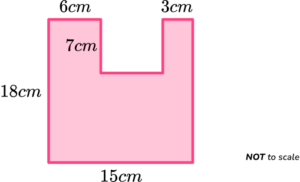49 \ cm270 \ cm66 \ cm80 \ cmThe missing sides are 6 \ cm , 7 \ cm and 18 \ cm.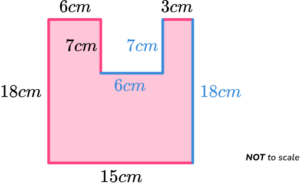The perimeter is 80 \ cm because

6+7+6+7+3+18+15+18=80.

6. The perimeter of this 2D shape is 48 \ m. Find the value of x.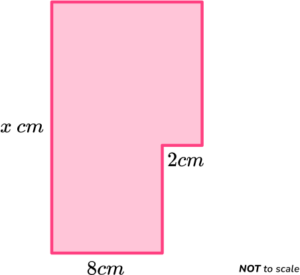12141618The missing horizontal side is 10 \ cm.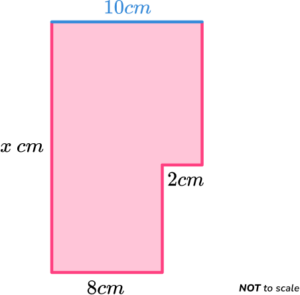The two vertical missing sides will add together to make x \ cm.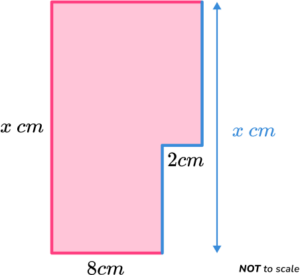The perimeter of the shape can be used to form an equation which can be solved to find x.

\begin{aligned} 2x+20 &= 48 \\\\ 2x &= 28 \\\\ x&=14 \end{aligned}

### Perimeter of compound shapes GCSE questions

1. Calculate the perimeter of the shape below.(2 marks)

For finding the missing sides.(1)

42 \ m

(1)

2. Calculate the perimeter of the shape below.(3 marks)

For finding the missing base, 10 \ cm.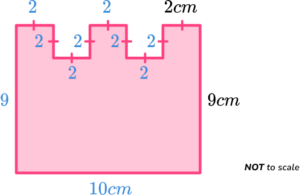(1)

(9 \times 2)+10+(2 \times 9)

(1)

46 \ cm

(1)

3. (a) Calculate the perimeter of the shape below.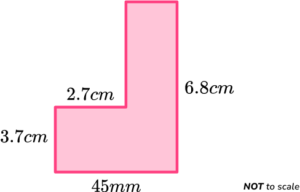(b) A regular pentagon has the same perimeter as the shape in part a.

Find the length of one side of the regular pentagon.

(5 marks)

(a)

45 \ mm = 4.5 \ cm \ (or 6.8 \ cm = 68 \ mm)

(1)

1.8 \ cm \ ( or 18 \ mm) and 3.1 \ cm ( or 31 \ mm).

(1)

Perimeter is 22.6 \ cm \ ( or 226 \ mm) .

(1)

(b)

22.6 \div 5 \ ( or 226 \div 5)

(1)

4.52 \ cm \ ( or 45.2 \ mm)

(1)

## Learning checklist

You have now learned how to:

• Work out the perimeter of compound shapes
• Find the missing side of a compound shape, given its perimeter
• Solve problems involving the perimeter of compound shapes (or composite shapes)

## Still stuck?

Prepare your KS4 students for maths GCSEs success with Third Space Learning. Weekly online one to one GCSE maths revision lessons delivered by expert maths tutors.

Find out more about our GCSE maths tuition programme.